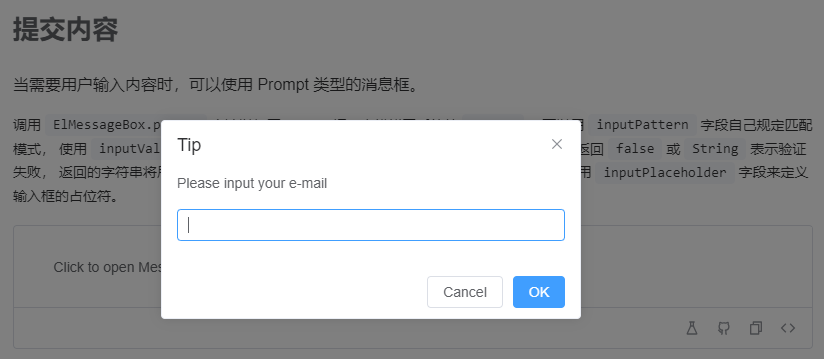# 对于前端开发，表单提交的最后一步该干些什么``````ElMessageBox.prompt('请输入内容', 'Tip', {
confirmButtonText: '确定',
cancelButtonText: '取消',
inputPattern: /^.+\$/,
inputErrorMessage: '输入内容不能为空',
})
.then(({ value }) => {
ElMessage({
type: 'success',
message: `输入的内容为:\${value}`,
})
})
.catch(() => {
ElMessage({
type: 'info',
message: '取消输入',
})
})
``````

``````ElMessageBox({
title: "提示",
message: () =>
h("div", {}, [
h(
"p",
{ style: { "margin-bottom": "10px" } },
"选择导出数据类型，默认只导出当前页的表格数据"
),
h(ElSwitch, {
modelValue: checked.value,
activeText: "全部",
activeValue: true,
inactiveText: "当前页",
inactiveValue: false,
"onUpdate:modelValue": (val: boolean) => {
checked.value = val;
}
})
])
})
.then(async () => {
if (checked.value) {
// todo
}
})
.catch(() => {
// todo
});
``````

``````let childEles = [];
const { Code, Data } = await getPrdLineInfo();
if (Code === 0 && Data.length) {
childEles = Data.map(el => {
if (el.IsSelected) {
formData.value.ProductLineId = el.PrdLineId;
}
return h(ElOption, {
value: el.PrdLineId,
label: el.PrdLineName
});
});
}
ElMessageBox({
title: "提示",
showCancelButton: true,
message: () =>
h("div", [
h(
"p",
{ style: { "margin-bottom": "10px" } },
message + "，确定要提交数据吗？"
),
h("p", { style: { "margin-bottom": "5px" } }, "选择产品线"),
h(
ElSelect,
{
modelValue: formData.value.ProductLineId,
"onUpdate:modelValue": (val: string) => {
formData.value.ProductLineId = val;
},
style: { width: "100%" }
},
() => childEles
)
])
})
.then(async () => {
// todo
})
.catch(() => {
// todo
});
``````

Sidebar